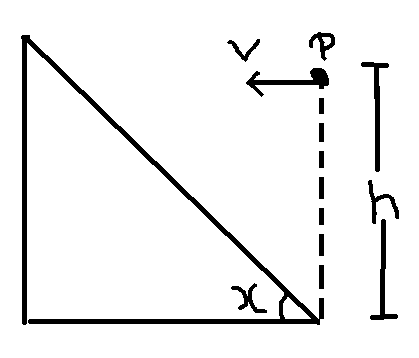# Recollect the past 3!

Determine the horizontal velocity $v$ with which a stone must be projected horizontally from a point $P$, so that it may hit the inclined plane perpendicularly. The inclination of the plane with the horizontal is $x$ and point $P$ is at a height $h$ above the foot of the incline.Details And assumptions

Take $g=10\frac{\text{m}}{\text{s}^2}$

$h = 10\text{ m}$

$x = {45}^{\circ}$

Round off the answer to the nearest integer.

×